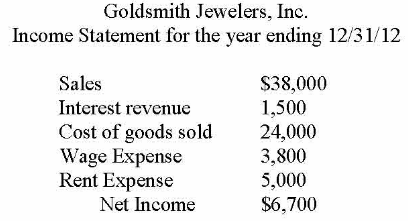# Problem: The 5,000 shares of Goldsmith's outstanding common stock originally sold for $10 per share. As of 12/31/12, the stock is selling at$15 per share. What is Goldsmith's price/earnings ratio as of 12/31/12 (round to 2 decimal places)? A. 3.73 B. 3.00 C. 11.19 D. 7.46

###### Problem Details

The 5,000 shares of Goldsmith's outstanding common stock originally sold for $10 per share. As of 12/31/12, the stock is selling at$15 per share. What is Goldsmith's price/earnings ratio as of 12/31/12 (round to 2 decimal places)?

A. 3.73

B. 3.00

C. 11.19

D. 7.46Frequently Asked Questions

What scientific concept do you need to know in order to solve this problem?

Our tutors have indicated that to solve this problem you will need to apply the Ratios: Price-Earnings Ratio (PE Ratio) concept. You can view video lessons to learn Ratios: Price-Earnings Ratio (PE Ratio). Or if you need more Ratios: Price-Earnings Ratio (PE Ratio) practice, you can also practice Ratios: Price-Earnings Ratio (PE Ratio) practice problems.

What is the difficulty of this problem?

Our tutors rated the difficulty ofThe 5,000 shares of Goldsmith's outstanding common stock ori...as medium difficulty.